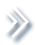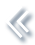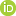####ArchivesReview Articles

## Combining estimators of a common parameter across samples

Eric Slud ,

Mathematics Department, University of Maryland College Park, College Park, MD, USA; Center for Statistical Research and Methodology, US Census Bureau, Washington DC, USAevs@math.umd.edu

Ilia Vonta ,

Dept. of Mathematics, National Technical University of Athens, Athens, GreeceAbram Kagan

Mathematics Department, University of Maryland College Park, College Park, MD, USAPages 158-171 | Received 21 Mar. 2018, Accepted 28 Sep. 2018, Published online: 24 Oct. 2018,
• Abstract
• References
• Citations

ABSTRACT

In many settings, multiple data collections and analyses on the same topic are summarised separately through statistical estimators of parameters and variances, and yet there are scientific reasons for sharing some statistical parameters across these different studies. This paper summarises what is known from large-sample theory about when estimators of a common structural parameter from several independent samples can be combined functionally, or more specifically linearly, to obtain an asymptotically efficient estimator from the combined sample. The main idea is that such combination can be done when the separate-sample nuisance parameters, if any exist, vary freely and independently of one another. The issues are illustrated using data from a multi-centre lung cancer clinical trial. Examples are presented to show that separate estimators cannot always be combined in this way, and that the functionally combined separate estimators may have low or 0 efficiency compared to the unified analysis that could be performed by pooling the datasets.

## References

1. Azzalini, A. (1985). A class of distributions which includes the normal ones. Scandinavian Journal of Statistics12, 171–178. [Google Scholar]
2. Bickel, P., & Doksum, K. (2007). Mathematical statistics (2nd ed., Vol. I). Upper Saddle River, NJ: Pearson Prentice Hall. updated printing. [Google Scholar]
3. Bickel, P., Klaassen, C., Ritov, Y., & Wellner, J. (1998). Efficient and adaptive estimation for semiparametric models. Berlin: Springer. [Google Scholar]
4. Carlin, B., & Louis, T. (2008). Bayesian methods for data analysis (3rd ed.). Boca Raton, FL: Chapman & Hall/CRC. [Crossref], [Google Scholar]
5. DeMissie, M. (2009). Investigating center effects in a multi-center clinical trial study using a parametric proportional hazards meta-analysis model (MS Thesis). Univ. of Maryland Statistics Program, DRUM Digital Repository. Retrieved from https://drum.lib.umd.edu/handle/1903/9588 [Google Scholar]
6. Draper, N., & Smith, H. (1981). Applied regression analysis (2nd ed.). New York: Wiley. [Google Scholar]
7. Efron, B. (1996). Empirical Bayes methods for combining likelihoods. Journal of the American Statistical Association91, 538–550. doi: 10.1080/01621459.1996.10476919 [Taylor & Francis Online], [Google Scholar]
8. Gray, R. (1994). A Bayesian analysis of institutional effects in a multicenter cancer clinical trial. Biometrics50, 244–253. doi: 10.2307/2533216 [Google Scholar]
9. Hartung, J., Knapp, G., & Sinha, B. (2008). Statistical meta-analysis with applications. Hoboken, NJ: Wiley. [Google Scholar]
10. Janicki, R. (2009). Statistical inference based on estimating functions in exact and misspecified models (Ph.D. thesis). Univ. of Maryland Statistics Program, DRUM Digital Repository. Retrieved from https://drum.lib.umd.edu/handle/1903/9690 [Google Scholar]
11. Kagan, A., & Rao, C. R. (2003). Some properties and applications of the efficient Fisher score. Journal of Statistical Planning & Inference116, 343–352. doi: 10.1016/S0378-3758(02)00351-8 [Google Scholar]
12. Lin, D. Y., & Zeng, D. (2010). On the relative efficiency of using summary statistics versus individual-level data in meta-analysis. Biometrika97, 321–332. doi: 10.1093/biomet/asq006 [Google Scholar]
13. Liu, D., Liu, R., & Xie, M. (2015). Multivariate meta-analysis of heterogeneous studies using only summary statistics: Efficiency and robustness. Journal of the American Statistical Association110, 326–340. doi: 10.1080/01621459.2014.899235 [Taylor & Francis Online], [Google Scholar]
14. Olkin, I., & Sampson, A. (1998). Comparison of meta-analysis versus analysis of variance of individual patient data. Biometrics54, 347–352. doi: 10.2307/2534018 [Google Scholar]
15. R Development Core Team (2017). R: A language and environment for statistical computing. Vienna: R Foundation for Statistical Computing. Retrieved from http://www.R-project.org, ISBN 3-900051-07-0. [Google Scholar]
16. Rao, C. R. (1973). Linear statistical inference (2nd ed.). New York: Wiley. [Google Scholar]
17. Rao, J. N. K., & Molina, I. (2015). Small area estimation (2nd ed.). Hoboken, NJ: Wiley. [Google Scholar]
18. Slud, E., & DeMissie, M. (2011). Validity of regression meta-analyses versus pooled analyses of mixed-effect linear models. Mathematics in Engineering, Science and Aerospace2(4), 251–266. [Google Scholar]
19. Tsiatis, A. (2006). Semiparametric theory and missing data. New York: Springer. [Google Scholar]
20. Van der Vaart, A. (1998). Asymptotic statistics. Cambridge: Cambridge University Press. [Google Scholar]